Courses

# Lagrangian and Hamiltonian Formalism and equations of motion (Part - 2) - CSIR-NET Physical Sciences Physics Notes | EduRev

## Physics : Lagrangian and Hamiltonian Formalism and equations of motion (Part - 2) - CSIR-NET Physical Sciences Physics Notes | EduRev

The document Lagrangian and Hamiltonian Formalism and equations of motion (Part - 2) - CSIR-NET Physical Sciences Physics Notes | EduRev is a part of the Physics Course Physics for IIT JAM, UGC - NET, CSIR NET.
All you need of Physics at this link: Physics

2.3 Hamilton’s Principle

The configuration of a system at any moment is speci ed by the value of the generalized coordinates q(t), and the space coordinatized by these q1,......,qN is the configuration space. The time evolution of the system is given by the tra jectory, or motion of the point in con guration space as a function of time, which can be speci ed by the functions qi(t).
One can imagine the system taking many paths, whether they obey Newton’s Laws or not. We consider only paths for which the qi(t) are differentiable. Along any such path, we de ne the action as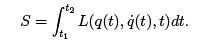(2.16)

The action depends on the starting and ending points q(t1) and q(t2), but beyond that, the value of the action depends on the path, unlike the work done by a conservative force on a point moving in ordinary space. In fact, it is exactly this dependence on the path which makes this concept useful | Hamilton’s principle states that the actual motion of the particle from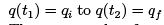is along a path q(t) for which the action is stationary.
That means that for any small deviation of the path from the actual one, keeping the initial and nal con gurations xed, the variation of the action vanishes to rst order in the deviation.

To find out where a differentiable function of one variable has a stationary point, we differentiate and solve the equation found by setting the derivative to zero. If we have a diff
erentiable function f of several variables xi, the first-order variation of the function is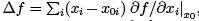so unless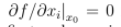for all i, there is some variation of the {xi} which causes a first order variation of f, and then x0 is not a stationary point.

But our action is a functional, a function of functions, which represent an in nite number of variables, even for a path in only one dimension. Intuitively, at each time q(t) is a separate variable, though varying q at only one point makes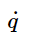hard to interpret. A rigorous mathematician might want to describe the path q(t) on t ∈ [0, 1] in terms of Fourier series, for which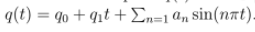Then the functional S(f) given by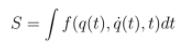becomes a function of the in nitely many variables q0,q1,a1,.....The endpoints x q0 and q1, but the stationary condition gives an in nite number of equations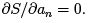It is not really necessary to be so rigorous, however. Under a change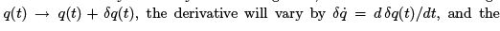functional S will vary by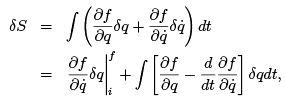where we integrated the second term by parts. The boundary terms each have a factor of δq at the initial or nal point, which vanish because Hamilton tells us to hold the qi and qf xed, and therefore the functional is stationary if and only if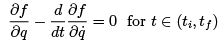(2.17)

We see that if f is the Lagrangian, we get exactly Lagrange’s equation. The above derivation is essentially unaltered if we have many degrees of freedom qi instead of just one.

2.3.1 Examples of functional variation

In this section we will work through some examples of functional variations both in the context of the action and for other examples not directly related to mechanics.

The falling particle

As a rst example of functional variation, consider a particle thrown up in a uniform gravitional eld at t = 0, which lands at the same spot at t = T .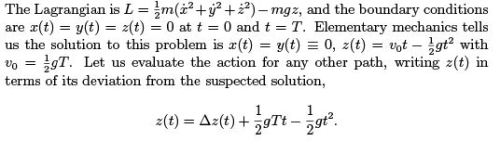We make no assumptions about this path other than that it is differentiable and meets the boundary conditions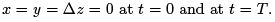The action is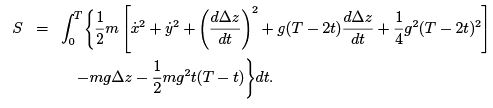The fourth term can be integrated by parts,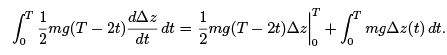The boundary term vanishes becamugs Δz = 0 where it is evaluated, and the other term cancels the sixth term in S ,so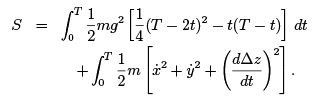The rst integral is independent of the path, so the minimum action requires the second integral to be as small as possible. But it is an integral of a nonnegative quantity, so its minimum is zero, requiring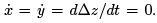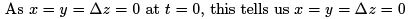at all times, and the path which minimizes the action is the one we expect from elementary mechanics.

Is the shortest path a straight line?

The calculus of variations occurs in other contexts, some of which are more intuitive. The classic example is to nd the shortest path between two points in the plane. The length ‘ of a path y(x) from (x1,y1)to(x2,y2) is given5 by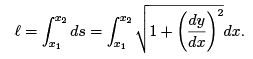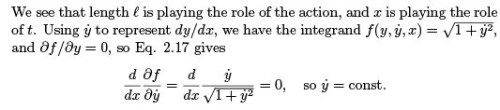and the path is a straight line.

2.4 Conserved Quantities

2.4.1 Ignorable Coordinates

If the Lagrangian does not depend on one coordinate, say qk , then we say it is an ignorable coordinate. Of course, we still want to solve for it, as its derivative may still enter the Lagrangian and effect the evolution of other coordinates. By Lagrange’s equation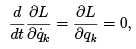so if in general we de ne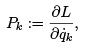as the generalized momentum, then in the case that L is independent of qk , Pk is conserved, dPk /dt =0.

Linear Momentum

As a very elementary example, consider a particle under a force given by a potential which depends only on y and z, but not x. Then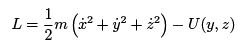is independent of x, x is an ignorable coordinate and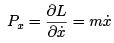is conserved. This is no surprize, of course, because the force is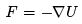and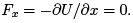Note that, using the definition of the generalized momenta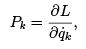Lagrange’s equation can be written as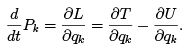Only the last term enters the de nition of the generalized force, so if the kinetic energy depends on the coordinates, as will often be the case, it is not true that  dPk =dt = Q. In that sense we might say that the generalized momentum and the generalized force have not been de ned consistently.

Angular Momentum

As a second example of a system with an ignorable coordinate, consider an axially symmetric system described with inertial polar coordinates (r, θ, z ), with z along the symmetry axis. Extending the form of the kinetic energy we found in sec (1.3.4) to include the z coordinate, we have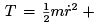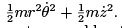The potential is independent of θ, because otherwise the system would not be symmetric about the z-axis, so the Lagrangian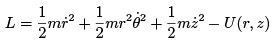does not depend on θ, which is therefore an ignorable coordinate, and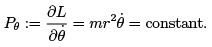We see that the conserved momentum Pθ is in fact the z-component of the angular momentum, and is conserved because the axially symmetric potential can exert no torque in the z -direction: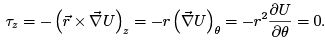Finally, consider a particle in a spherically symmetric potential in spherical coordinates. In section (3.1.2) we will show that the kinetic energy in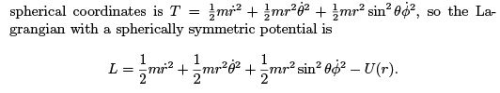Again, φ is an ignorable coordinate and the conjugate momentum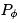is conserved. Note, however, that even though the potential is independent of θ as well, θ does appear undifferentiated in the Lagrangian, and it is not an ignorable coordinate, nor is Pθ conserved.

If qj is an ignorable coordinate, not appearing undifferentiated in the Lagrangian, any possible motion qj (t) is related to a different tra jectory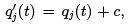in the sense that they have the same action, and if one is an extremal path, so will the other be. Thus there is a symmetry of the system under qj → q+ c, a continuous symmetry in the sense that c can take on any value. As we shall see in Section 8.3, such symmetries generally lead to conserved quantities. The symmetries can be less transparent than an ignorable coordinate, however, as in the case just considered, of angular momentum for a spherically symmetric potential, in which the conservation of Lz follows from an ignorable coordinate φ, but the conservation of Lx and Ly follow from symmetry under rotation about the x and y axes respectively, and these are less apparent in the form of the Lagrangian.

2.4.2 Energy Conservation

We may ask what happens to the Lagrangian along the path of the motion.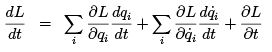In the first term the first factor is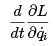by the equations of motion, so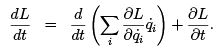We expect energy conservation when the potential is time invariant and there is not time dependence in the constraints, i.e. when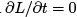, so we rewrite this in terms of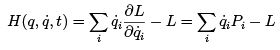Then for the actual motion of the system,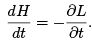If, H is conserved.

H is essentially the Hamiltonian, although strictly speaking that name is reserved for the function H (q, p, t) on extended phase space rather than the function with arguments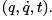. What is H physically? In the case of Newtonian mechanics with a potential function, L is an inhomogeneous quadratic function of the velocities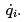. If we write the Lagrangian L = L2 + L1 + L0 as a sum of pieces purely quadratic, purely linear, and independent of the velocities respectively, then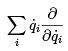is an operator which multiplies each term by its order in velocities,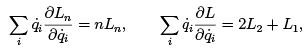and

H = L2 − L0,
For a system of particles described by their cartesian coordinates, L2 is just the kinetic energy T , while L0 is the negative of the potential energy L0 = −U ,so H = T + U is the ordinary energy. There are, however, constrained systems, such as the bead on a spoke of Section 2.2.1, for which the Hamiltonian is conserved but is not the ordinary energy.

Hamilton’s Equations

We have written the Lagrangian as a function of qi,, and t, soitisa function of N + N + 1 variables. For a free particle we can write the kinetic energy either as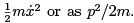More generally, we can7 reexpress the dynamics in terms of the 2N + 1 variables qk , P, and t.

The motion of the system sweeps out a path in the spaceora path in (q, P, t). Along this line, the variation of L is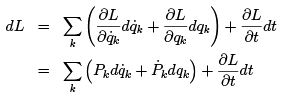where for the rst term we used the de nition of the generalized momentum and in the second we have used the equations of motion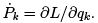Then examining the change in the Hamiltonian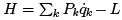along this actual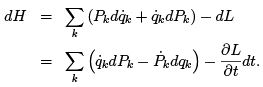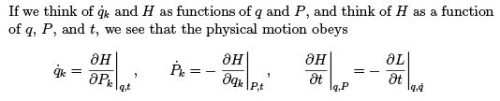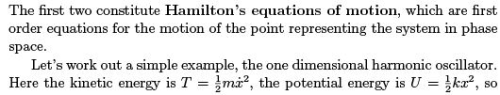2.5. HAMILTON’S EQUATIONS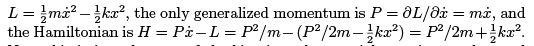Note this is just the sum of the kinetic and potential energies, or the total energy.

Hamilton’s equations give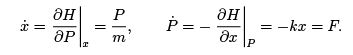These two equations verify the usual connection of the momentum and velocity and give Newton’s second law.
The identi cation of H with the total energy is more general than our particular example. If T is purely quadratic in velocities, we can write T =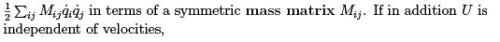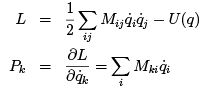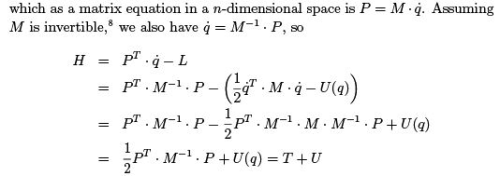so we see that the Hamiltonian is indeed the total energy under these circumstances.

Offer running on EduRev: Apply code STAYHOME200 to get INR 200 off on our premium plan EduRev Infinity!

159 docs

,

,

,

,

,

,

,

,

,

,

,

,

,

,

,

,

,

,

,

,

,

;